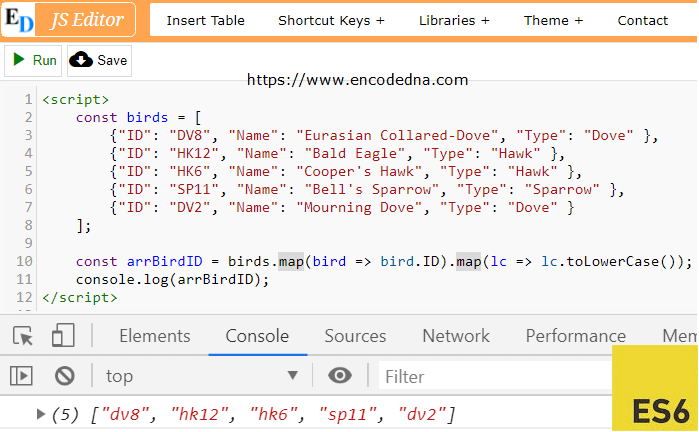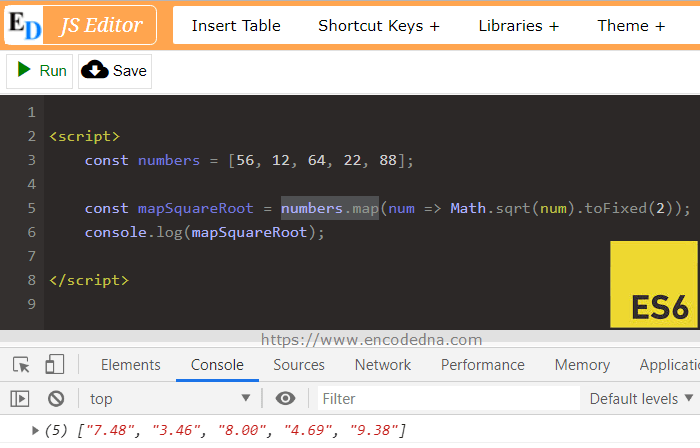﻿ ES6 map() function Example

# ES6 map() function Example

The map() function creates a new array from another array. It uses the same elements from the parent array and creates a new array of elements by calling a function. In many different ways you can use the map() function in JavaScript. I am sharing few examples here.

## Syntax of map() function

array.map (function callback)

The map() function iterates or loops through an array using a callback function (or the mapping function). See the syntax. Each element is sent to the callback function, which then returns a new element, and the map() creates a new array using the returned elements.

Example 1

In the first example, I have an array of code and each code is an alpha-numeric value. I want to extract only the text values (alphabets) and create a new array out of it.

```<html>
<title>ES6 map() function Example</title>
<body>
<h2>Get only text from array using map()</h2>
<p id="msg"></p>
</body>
<script>
const arrCode = ['a1', 'c21', 'b9', 'cq87', 'qi8'];

const getOnlyText = (val) => {
return val.match(/\D+/g);
}

let a_new_array = arrCode.map(getOnlyText);

let msg = document.getElementById('msg');
msg.innerHTML = a_new_array;
</script>
</html>```

In the above example, the callback function (getOnlyText ()) takes a value as parameter. The array map() calls the function using one element (from the parent array "arrCode []") at a time and the callback function returns the text value.

Note: The map(), internally loops through each element in the array. It actually spares us from using a for loop or even functions such as the .forEach() or for (… of), to achieve our goal.

In-addition, see how I have used ES6’s arrow function (=>), with my function. The arrow function is another key feature, which was introduced in ES6.

const getOnlyText = (val) => { }

Example 2

In this second example, I am using the map() function against a JSON array, to create a new array. The JSON has three objects, the id, name and type of birds.

Using the map() I can filter out the elements of my choice from the array.

```<html>
<body>
<p id="msg"></p>
</body>
<script>
const birds = [
{"ID": "DV8", "Name": "Eurasian Collared-Dove", "Type": "Dove" },
{"ID": "HK12", "Name": "Bald Eagle", "Type": "Hawk" },
{"ID": "HK6", "Name": "Cooper's Hawk", "Type": "Hawk" },
{"ID": "SP11", "Name": "Bell's Sparrow", "Type": "Sparrow" },
{"ID": "DV2", "Name": "Mourning Dove", "Type": "Dove" }
];

const arrBirdID = birds.map(bird => bird.ID);	     // Or, simply use bird.Name to get the values from the "Name" object in the JSON array.
document.getElementById('msg').innerHTML = arrBirdID;
</script>
</html>```

See how I have used the map() function in this example. That's another way you can use the map() function on an array. And, again, I have used the arrow (=>) function to shorten my code.

This one-liner code (in the above example) is actually a simplified alternative of this method ...

```const bird = (val) => {
return val.ID;
}
const arrBirdID = birds.map(bird);```

Now try this ...

You can use multiple map() functions in a single statment. For example,

```const arrBirdID = birds.map(bird => bird.ID).map(lc => lc.toLowerCase());
console.log(arrBirdID);
```

This is like saying, map all the IDs and convert it into lower case>. See the bottom of the image.

imageExample 3

Here’s another example.

```<script>
const numbers = [56, 12, 64, 22, 88];

const square = (val) => {
return Math.sqrt(val).toFixed(2);
}

const mapSquareRoot = numbers.map(square);
console.log(mapSquareRoot);

//   OR SIMPLY USE ...

//  const numbers = [56, 12, 64, 22, 88];
//  const mapSquareRoot = numbers.map(num => Math.sqrt(num).toFixed(2));
//  console.log(mapSquareRoot);  ```In the above example, the map() creates a new array of elements, which is the square root of each number in the parent array. To get the square root, I am using the pre-defined Math.sqrt() method.

Conclusion

The map() serves as an alternative to your for loops. It reduces the length of your code and all it needs is a little practice. I have learned few tricks using this amazing ES6 feature and now, you too know how to use it.

Don't forget that you can use multiple map() functions in a single statement.